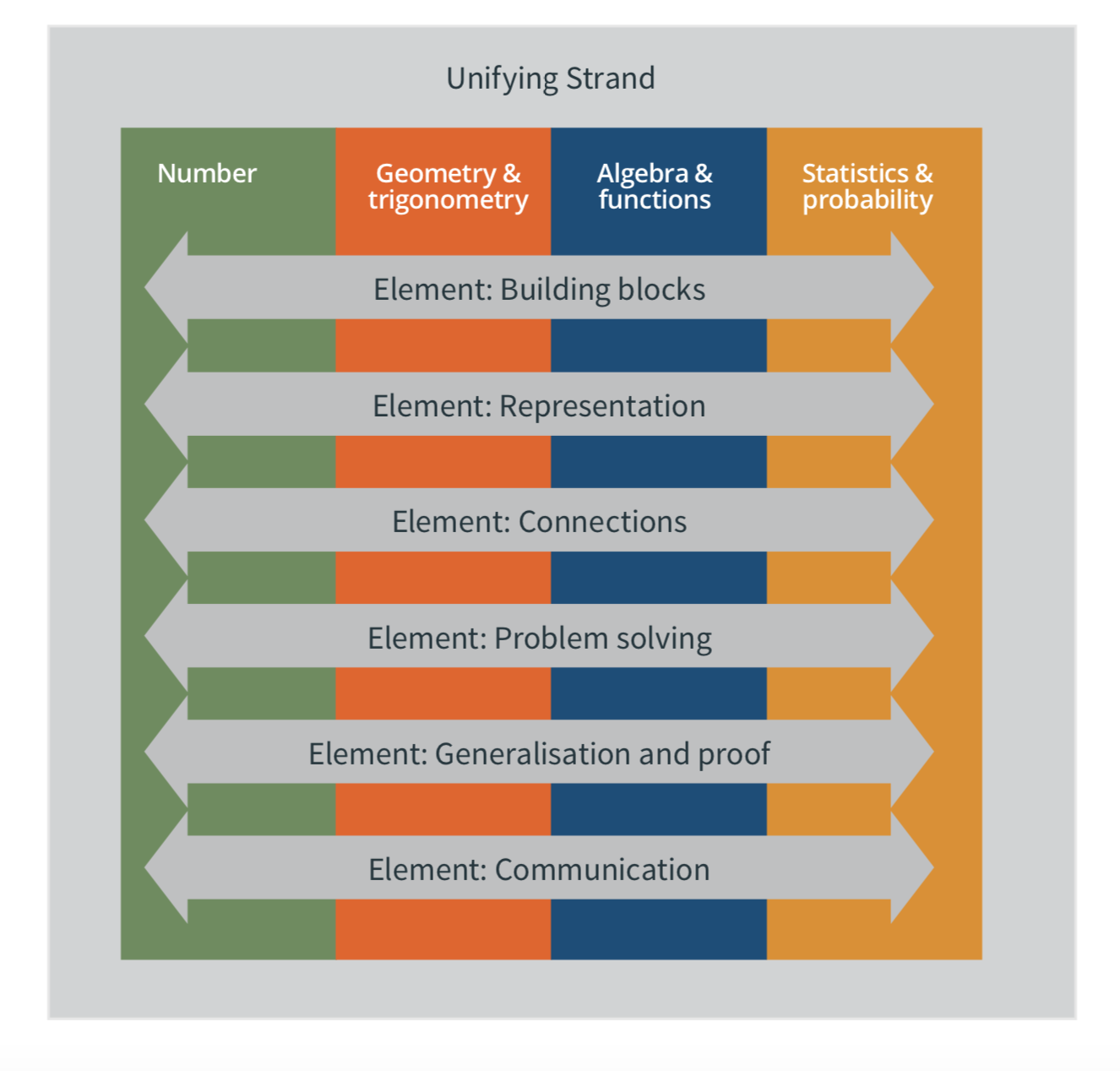# Overview: Course

The specification for junior cycle mathematics focuses on developing students’ ability to think logically, strategically, critically, and creatively through the Unifying strand and the four contextual strands: Number; Geometry and trigonometry; Algebra and functions; and Statistics and probability.
The specification has been designed for a minimum of 240 hours timetabled student engagement across the three years of junior cycle. This is a minimum and schools should be aware that there are students who would benefit from an engagement of more than than 240 hours to realise the national improvement targets set out in the  Literacy and Numeracy strategy (DES,2011).

##### Figure 2: The structure of the specification for junior cycle mathematics## Unifying strand

This strand permeates all of the contextual strands and is composed of the six elements of the specification, which are shown below.
There is no specific content linked to this strand; rather, its learning outcomes underpin the rest of the specification. Each learning outcome in this strand is applicable to all of the activities and content of the other four strands—for example, students should be able to draw on all of their mathematical knowledge and skills to solve a problem or to communicate mathematics.
Furthermore, the elements of this strand are interdependent, so that students should develop the different skills associated with each element in tandem rather than in isolation – for example, engaging in problem-solving can help students improve their understanding of building blocks and their ability to make connections within mathematics.

Elements

Building blocks
Students should understand and recall the concepts that underpin each strand, and be able to carry out the resulting procedures accurately, effectively, and appropriately.
Representation Students should be able to represent a mathematical situation in a variety of different ways and translate flexibly between them.
Connections Students should be able to make connections within strands and between strands, as well as connections between mathematics and the real world.
Problem solving  Students should be able to investigate patterns, formulate conjectures, and engage in tasks in which the solution is not immediately obvious, in familiar and unfamiliar contexts.
Generalisation and proof Students should be able to move from specific instances to general mathematical statements, and to present and evaluate mathematical arguments and proofs.
Communication  Students should be able to communicate mathematics effectively in verbal and written form.

## Number

This strand focuses on different aspects of number, laying the groundwork for the transition from arithmetic to algebra. Learners explore different representations of numbers and the connections between them, as well as the properties and relationships of binary operations. They investigate number patterns, and use ratio and proportionality to solve a variety of problems in numerous contexts. Learners are expected to be able to use calculators appropriately and accurately, as well as to carry out calculations by hand and mentally. They appreciate when it is appropriate to use estimation and approximation, including to check the reasonableness of results.

## Geometry and trigonometry

This strand focuses on analysing characteristics and properties of two- and three-dimensional geometric shapes. Learners use geometry and trigonometry to model and solve problems involving area, length, volume, and angle measure. They develop mathematical arguments about geometric relationships and explore the concept of formal proof, using deduction to establish the validity of certain geometric conjectures and critiquing the arguments of others.

## Algebra and functions

This strand focuses on representing and analysing patterns and relationships found in numbers. Building on their work in the Number strand, learners generalise their observations, expressing, interpreting, and justifying general mathematical statements in words and in symbolic notation. They use the idea of equality to form and interpret equations, and the syntactic rules of algebra to transform expressions and solve equations. Learners explore and analyse the relationships between tables, diagrams, graphs, words, and algebraic expressions as representations of functions.

## Statistics and probability

This strand focuses on determining probability from random events and generating and investigating data. Students explore the relationship between experimental and theoretical probability as well as completing a data investigation; from formulating a question and designing the investigation through to interpreting their results in context and communicating their findings. Learners use graphical and numerical tools, including summary statistics and the concepts and processes of probability, to explore and analyse patterns in data. Through these activities, learners gain an understanding of data anaysis as a tool for learning about the world.